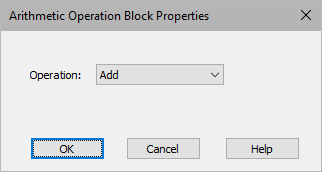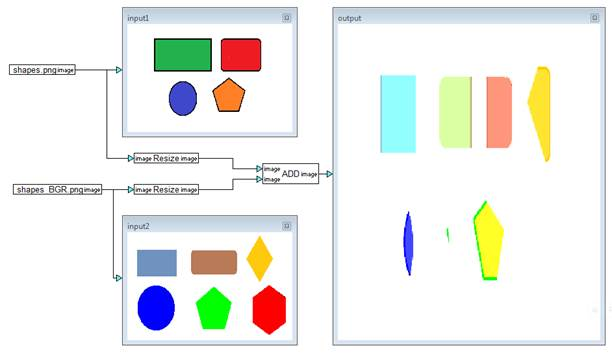### Arithmetic Operation

Block Category: Operations

Input Image Requirements: Must be same size and type

Description: The Arithmetic Operation block performs the specified arithmetic operation on each pixel value in two input images. The operation can be add, subtract, multiply, or divide. These operations are saturated operations. That is, if the operation produces a pixel value greater than 255, it is limited to 255; similarly, if the operation produces a pixel value less than 0, it is limited to 0.Operation: Specifies the operation to be performed on the input images.

#### Example

In this example, the results of an Add operation is displayed.